# Boyle's LawPage 1

#### WATCH ALL SLIDES

Slide 1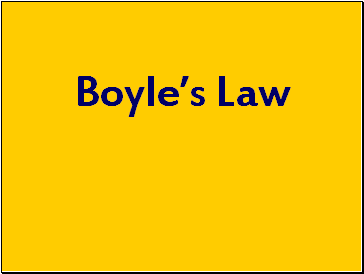Boyle’s Law

Slide 2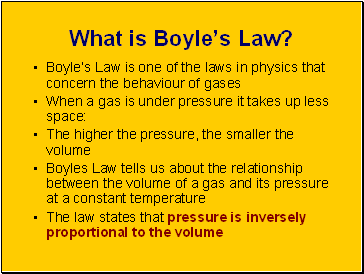## What is Boyle’s Law?

Boyle’s Law is one of the laws in physics that concern the behaviour of gases

When a gas is under pressure it takes up less space:

The higher the pressure, the smaller the volume

Boyles Law tells us about the relationship between the volume of a gas and its pressure at a constant temperature

The law states that pressure is inversely proportional to the volume

Slide 3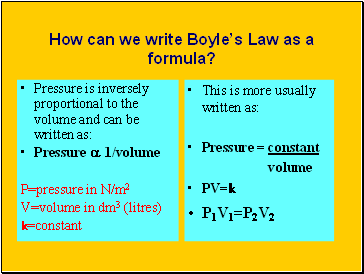## How can we write Boyle’s Law as a formula?

Pressure is inversely proportional to the volume and can be written as:

Pressure a 1/volume

P=pressure in N/m2

V=volume in dm3 (litres)

k=constant

This is more usually written as:

Pressure = constant

volume

PV=k

P1V1=P2V2

Slide 4## How can we investigate Boyle’s Law?

When investigating Boyles law a given volume of gas is sucked into a cylinder and the end is sealed

The temperature of the gas is kept constant

Using several equal weights we can apply increasing pressure to the gas

We can calculate the pressure by dividing the force applied by the area of the top of the cylinder

The volume will be shown on the scale on the cylinder

Slide 5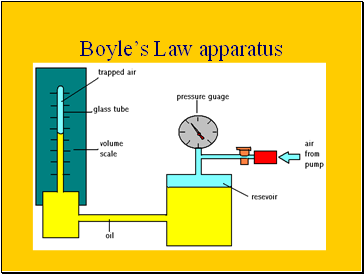## Boyle’s Law apparatus

Slide 6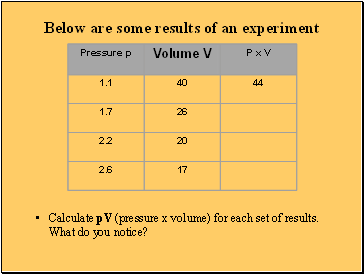## Below are some results of an experiment

Calculate pV (pressure x volume) for each set of results. What do you notice?

Slide 7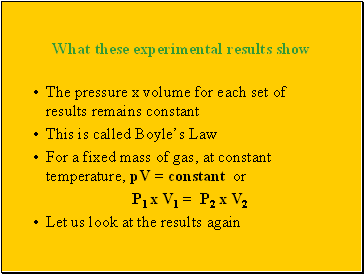## What these experimental results show

The pressure x volume for each set of results remains constant

This is called Boyle’s Law

For a fixed mass of gas, at constant temperature, pV = constant or

P1 x V1 = P2 x V2

Let us look at the results again

Slide 8Here are the results of the experiment

Did you notice that if p is doubled, V is halved?

If p increases to 3 times as much, V decreases to a 1/3rd . This means:

Volume is inversely proportional to pressure, or

V  1

p

Slide 9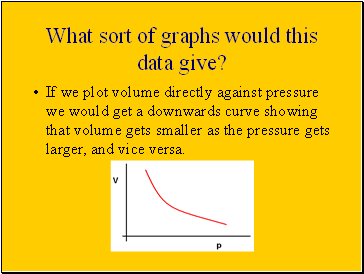What sort of graphs would this data give?

If we plot volume directly against pressure we would get a downwards curve showing that volume gets smaller as the pressure gets larger, and vice versa.

Slide 10Go to page:
1  2